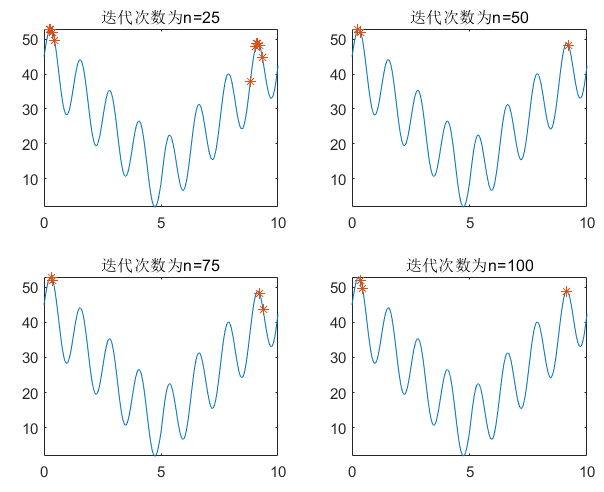## 什么是遗传算法

遗传算法，也叫Genetic Algorithm，简称 GA 算法他既然叫遗传算法，那么遗传之中必然有基因，那么基因染色体(Chromosome)就是它的需要调节的参数。我们在生物中了解到，大自然的法则是“物竞天择，适者生存”，遗传算法更适用于“优胜劣汰”。

• 优：最优解，
• 劣：非最优解。

## 遗传算法名词解释

下面主要通过疑问提问题的方式进行解释遗传算法当中的适应度函数、选择、交叉、变异这几个名词。

1. 都知道优胜劣汰，那怎么实现优胜劣汰呢？
很重要的一个环节就是选择，也称之为“大自然的选择”，大自然怎么选择呢，大自然会抛弃掉一些适应能力差的，在程序当中就是离最优解较远的解，也就是适应度低的解，会被抛弃掉。

2. 如何实现大自然的选择呢？
这里我们会引入轮盘赌法，进行大自然的选择。选择一些离最优解较近的个体。还有一些其他的经典的选择办法，例如锦标赛法进行选择。

3. 只靠选择就可以实现吗？那样会不会陷入局部最优解？
如果只靠选择进行调优，那么最终的结果会受到初始种群的影响，只是在初始种群的群体中进行选择，得出的最优解也是在初始群体中的最优解。所以就需要引入大自然当中 交叉。两个优秀的基因进行交叉可以将两者优秀的基因遗传给一代，也增加了群体的基因多样性，但这种不一定就是最好的，也可能发生“夭折”。

4. 大自然的选择好像不仅如此，还有变异吧？
为了更好的模拟大自然的选择规律，来需要进入变异，变异发生的概率很低，但也是增加群体多样性的条件，和交叉相同，变异求解出来的不一定是最好的解，也会出现“夭折”。

5. 上面只是大自然的规则，那么大自然的环境又是什么呢?
优秀的基因并不是独立的，就像北极熊不会存活在热带雨林一样。只有适合环境的基因才是优秀的，所以说基因具有相对性，环境是挑选基因的先决条件，这里的环境就是适应度函数。个体用过适应度函数后得到的结果越大，表明更加适合这里的环境，那么保留下来的概率越大。反之则越小。

## 遗传算法的程序实现

下面结合代码来梳理遗传算法的实现。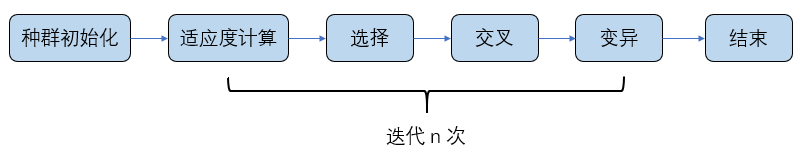涉及到还是适应度函数、选择、交叉、变异这几个模块。下面就这几个模块展开说明。具体的流程图解释如下：

1. 需要先对初始种群进行一次适应度函数进行计算，这样方便我们对个体进行选择，适应度值越大的越容易被保留；
2. 对群体进行选择，选择出适应度值较大的一部分优势群体；
3. 对优势种群进行 “交配”，更容易产生优秀的个体；
4. 模拟大自然变异操作，对染色体个体进行变异操作；

$f(x)=10\times sin(5\times x)+7\times \left|x-5\right|+10; x\in[0, 10]$

### 种群初始化

种群的初始化相对简单，只需要随机生成一个二维矩阵，矩阵的size=(种群大小，染色体编码长度)。染色体编码采用二进制编码的方式。染色体编码并不是直接采用将[0, 10]直接转换为二进制，原因如下：

• 并非均匀分布，10的二进制表示为1010，会导致出现空余位置，例如11、12等没有意义的数字出现
• 精度低，如果直接编码最小增量单位变成了 1 ，没有了浮点数的表示，最优解很多情况都会出现在浮点数的表示范围。n：代表染色体编码的长度

$x = \frac{染色体编码对应的十进制值}{2^n - 1}$

此时x的范围为[0, 1],我们可以根据待测得x轴的范围进行偏移计算。例如：x得范围为[2, 10],则设计:

$x = \frac{染色体编码对应的十进制值}{2^n - 1} \times 8 + 2$

种群初始化基本结构如下，实数范围还需要进一步计算得到真正得x轴的浮点值。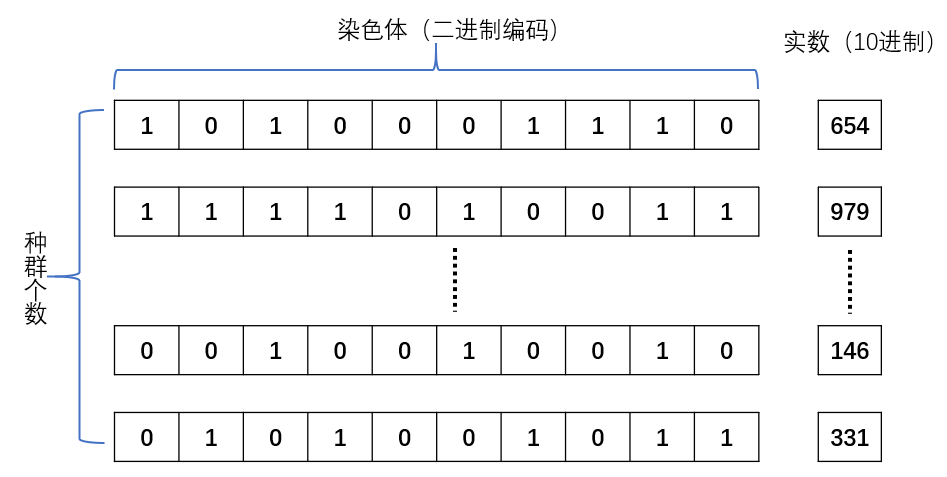%初始化种群大小
%输入变量：
%popsize：种群大小
%chromlength：染色体长度-->>转化的二进制长度
%输出变量：
%pop：种群
function pop=initpop(popsize,chromlength)
% round:产生的随机数进行四舍五入操作就是0或者1
pop = round(rand(popsize,chromlength));
### 适应度函数设计   适应度函数得出的值越大表明个体越优秀，所以一般情况下，在求解函数最大值的时候，适应度函数就是求解函数本身，求解最小值的时候适应度函数就是函数的倒数。在本例中求取最大值，所以适应度函数就是函数本身。
%二进制转化成十进制函数
%输入变量：
%二进制种群
%输出变量
%十进制数值
function pop2 = binary2decimal(pop)
[px,py]=size(pop); %取出pop的行数和列数
for i = 1:py %对于每一行
pop1(:,i) = 2.^(py-i).*pop(:,i); %每一行的二进制每一位进行换算
end
%sum(.,2)对行求和，得到列向量，每一个值就是该个体的十进制的值
temp = sum(pop1,2);
pop2 = temp*10/1023; %限制到[0, 10]

%计算函数目标值
%输入变量：二进制数值
%输出变量：目标函数值
function [objvalue] = cal_objvalue(pop)
x = binary2decimal(pop);
%转化二进制数为x变量的变化域范围的数值
objvalue=10*sin(5*x)+7*abs(x-5)+10;
### 选择   我们在前面已经说明了选择的原因（挑选优秀个体），挑选的算法有很多，我们这里选择“轮盘赌法”。轮盘赌法就是类似于我们玩的转盘，圆心角度越大的我们越容易选中。 **注：** 选择后后的个体数目和原种群个数相同。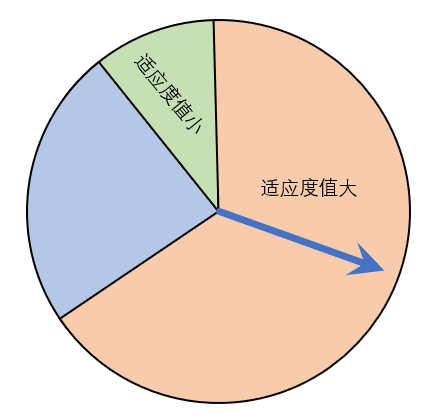大自然就像那个指针，适应度值越大，表明这个体约适应这个环境，那么被选择的概率越大，与此同时，适应度值小的个体并不代表不会被选中，只是选中的概率小，一方面也保证了正负样本的一个均衡。

%输出变量：newpop选择以后的二进制种群
function [newpop] = selection(pop,fitvalue)
%构造轮盘
[px,py] = size(pop);
totalfit = sum(fitvalue);
p_fitvalue = fitvalue/totalfit;
p_fitvalue = cumsum(p_fitvalue); % 数组各行累加值 （转盘）
% ms相当于指针，得出落在哪一个区域，就表明这个基因被选中
ms = sort(rand(px,1)); % 从小到大排列（指针）
fitin = 1; % 适应度矩阵的索引
newin = 1; % 新种群索引
while newin<=px
if(ms(newin))<p_fitvalue(fitin) % 表明落在了这一个区域
newpop(newin,:)=pop(fitin,:);
newin = newin+1;
else
fitin=fitin+1;
end
end

### 交叉

交叉相对来说，比较简单，就是将两个染色体进行基因片段的交叉。交叉的方式有很多，可以单个点进行交叉，随机基因片段交叉，随机长度的交叉方式，在这里仅实现随机长度的交叉方式。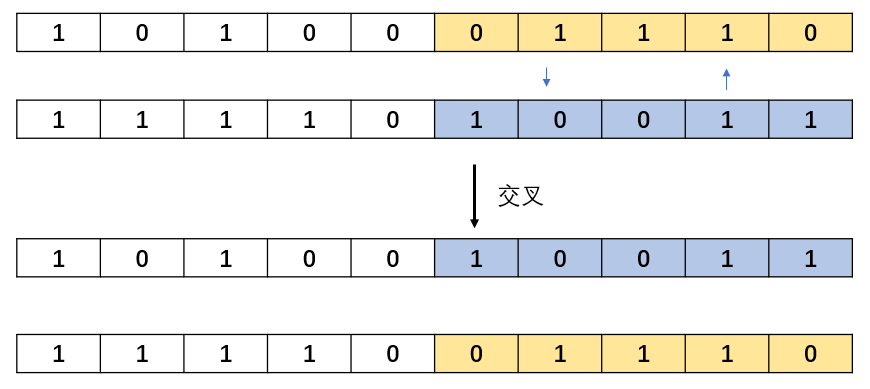%交叉变换（随机距离交叉）
%输入变量：pop：二进制的父代种群数，pc：交叉的概率
%输出变量：newpop：交叉后的种群数
function [newpop] = crossover(pop,pc)
[px,py] = size(pop);
newpop = ones(size(pop));
% 每两个进行一次交叉
for i = 1:2:px-1
if(rand<pc)
% 随机产生分割点
cpoint = round(rand*py);
% 交换位置
newpop(i,:) = [pop(i,1:cpoint),pop(i+1,cpoint+1:py)];
newpop(i+1,:) = [pop(i+1,1:cpoint),pop(i,cpoint+1:py)];
else
newpop(i,:) = pop(i,:);
newpop(i+1,:) = pop(i+1,:);
end
end

### 变异

变异就变得更加简单，对于二进制编码，只需要将随机一个基因位置进行取反操作。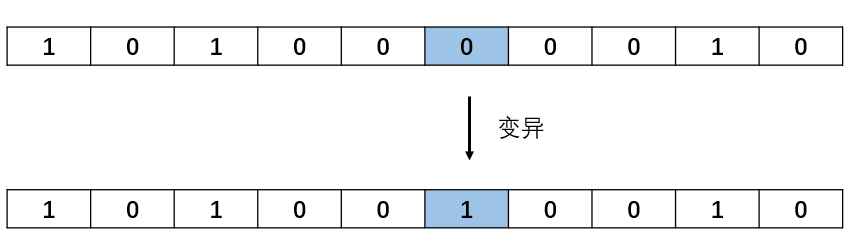%函数说明
%输入变量：pop：二进制种群，pm：变异概率
%输出变量：newpop变异以后的种群
function [newpop] = mutation(pop,pm)
[px,py] = size(pop);
newpop = ones(size(pop));
for i = 1:px
if(rand<pm)
mpoint = round(rand*py); % 变异位置
if mpoint <= 0
mpoint = 1;
end
newpop(i,:) = pop(i,:);
if newpop(i,mpoint) == 0  % 取反操作
newpop(i,mpoint) = 1;
else newpop(i,mpoint) == 1
newpop(i,mpoint) = 0;
end
else newpop(i,:) = pop(i,:);
end
end

### 主函数clear;
clc;
% 总种群数量
popsize=100;
% 染色体长度
chromlength=10;
% 交叉概率
pc = 0.6;
% 变异概率
pm = 0.001;
%初始化种群
pop = initpop(popsize,chromlength);  % 100 * 10

for i = 1:100
objvalue = cal_objvalue(pop);
fitvalue = objvalue;
newpop = selection(pop,fitvalue);
newpop = crossover(newpop,pc);
newpop = mutation(newpop,pm);
pop = newpop;
[bestindividual,bestfit] = best(pop,fitvalue);
x2 = binary2decimal(bestindividual);  % 最优解 x 值
x1 = binary2decimal(newpop);
y1 = cal_objvalue(newpop);
if mod(i,20) == 0
figure;
fplot(@(x)10*sin(5*x)+7*abs(x-5)+10,[0 10]);
hold on;
plot(x1,y1,'*');
title(['迭代次数：%d' num2str(i)]);
end
end
fprintf('The best X is --->>%5.2f\n',x2);
fprintf('The best Y is --->>%5.2f\n',bestfit);

## 运行结果展示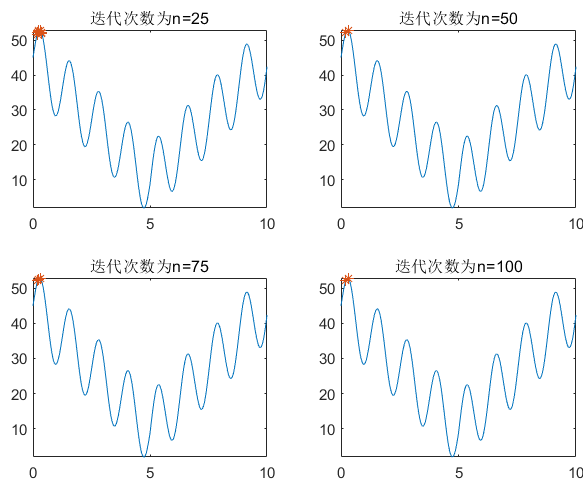## 总结